# Chapter 23 Electric Potential Electric Potential Energy Electric

• Slides: 48Chapter 23 Electric Potential & Electric Potential EnergyElectric Potential (Voltage) Electric potential is a scalar (not a vector). It can be positive or negative.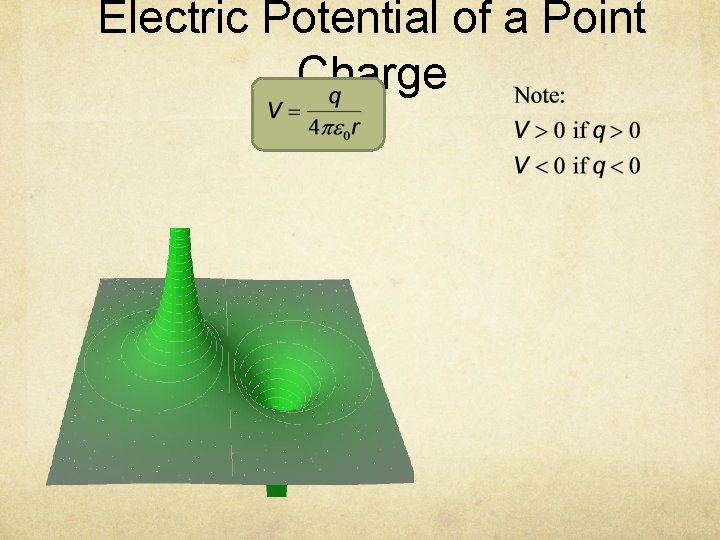Electric Potential of a Point Charge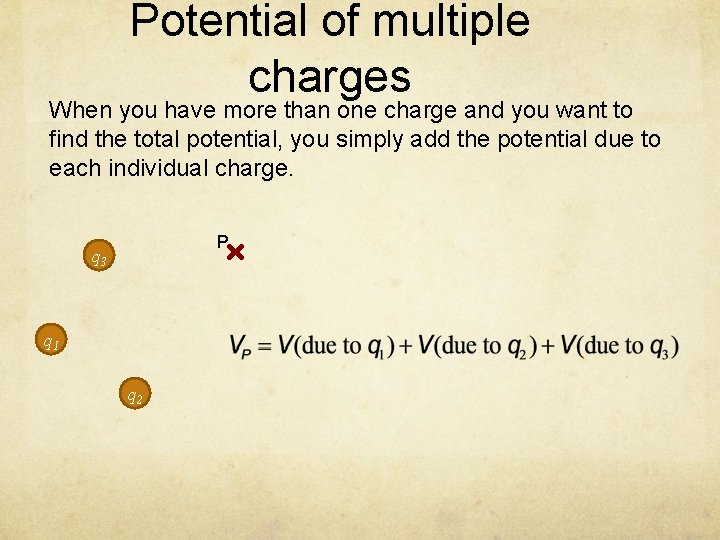Potential of multiple charges When you have more than one charge and you want to find the total potential, you simply add the potential due to each individual charge. P q 3 q 1 q 2Point charge potential A 1. 00 μC point charge is located at the origin and a second point charge of -4. 00 μC is located on the x-axis. Find the total electric potential at P due to these charges. y P 3. 00 m q 1 4. 00 m q 2 xPoint charge potential (The Faster Way) A 1. 00 μC point charge is located at the origin and a second point charge of -4. 00 μC is located on the x-axis. Find the total electric potential at P due to these charges. y P 3. 00 m q 1 4. 00 m q 2 x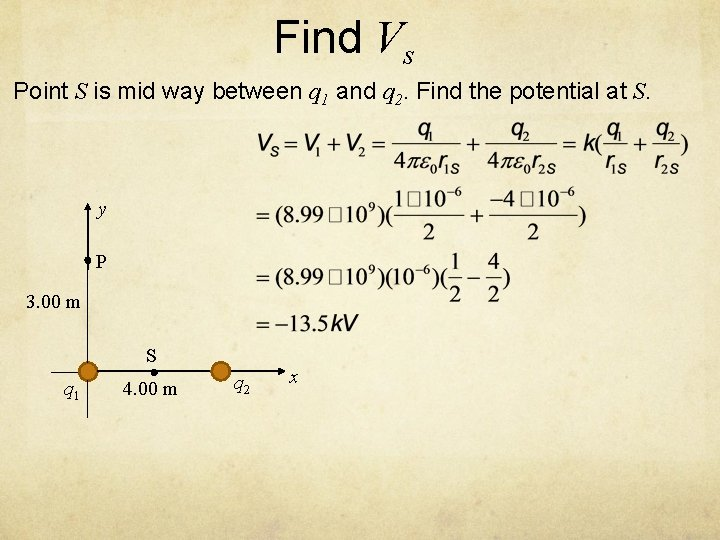Find Vs Point S is mid way between q 1 and q 2. Find the potential at S. y P 3. 00 m S q 1 4. 00 m q 2 x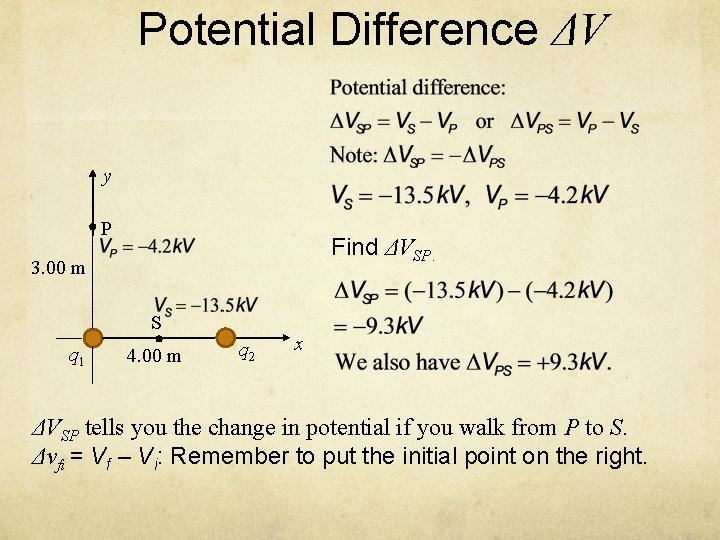Potential Difference ΔV y P Find ΔVSP. 3. 00 m S q 1 4. 00 m q 2 x ΔVSP tells you the change in potential if you walk from P to S. Δvfi = Vf – Vi: Remember to put the initial point on the right.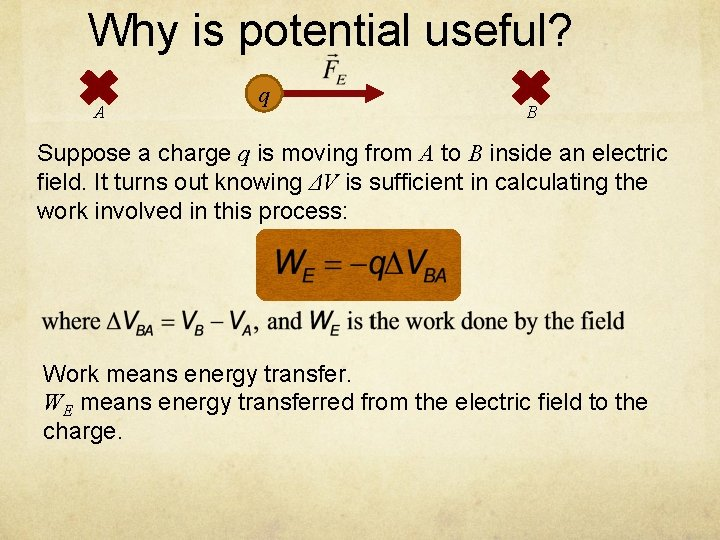Why is potential useful? A q B Suppose a charge q is moving from A to B inside an electric field. It turns out knowing ΔV is sufficient in calculating the work involved in this process: Work means energy transfer. WE means energy transferred from the electric field to the charge.The meaning of work Work means energy transfer. WE means energy transferred from the electric field to the charge. For example, if WE=100 J, it means the electric field has transferred 100 J of energy to the charge. If the charge is moving in free space (with no forces other than the electric force), then the energy gained by the charge becomes its KE. In real life applications, this energy from the electric field is extracted to power your appliances.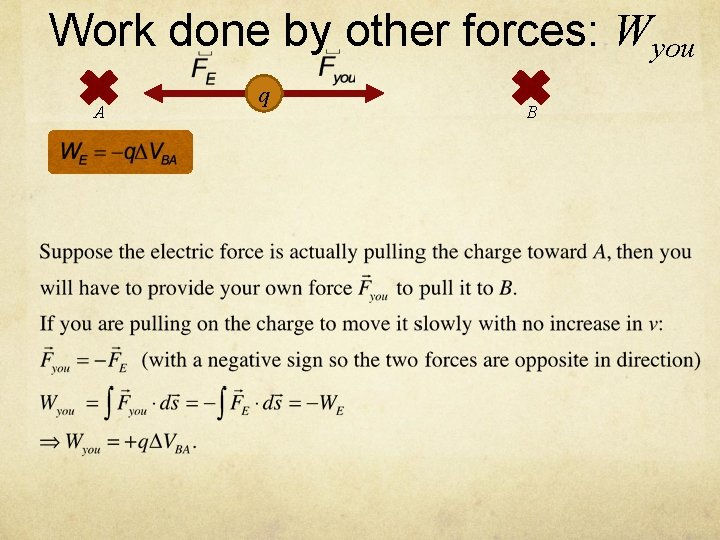Work done by other forces: Wyou A q B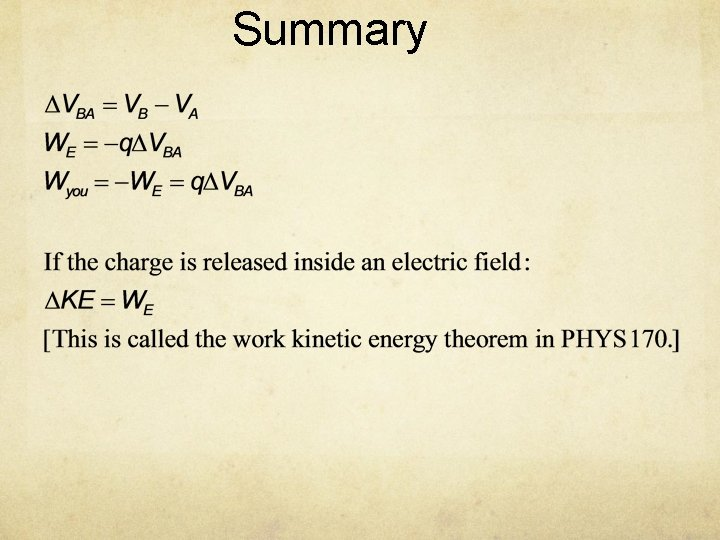Summary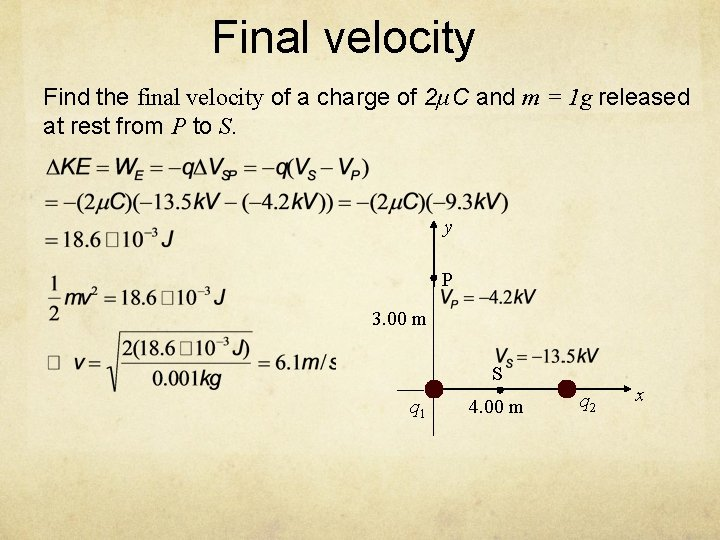Final velocity Find the final velocity of a charge of 2μC and m = 1 g released at rest from P to S. y P 3. 00 m S q 1 4. 00 m q 2 x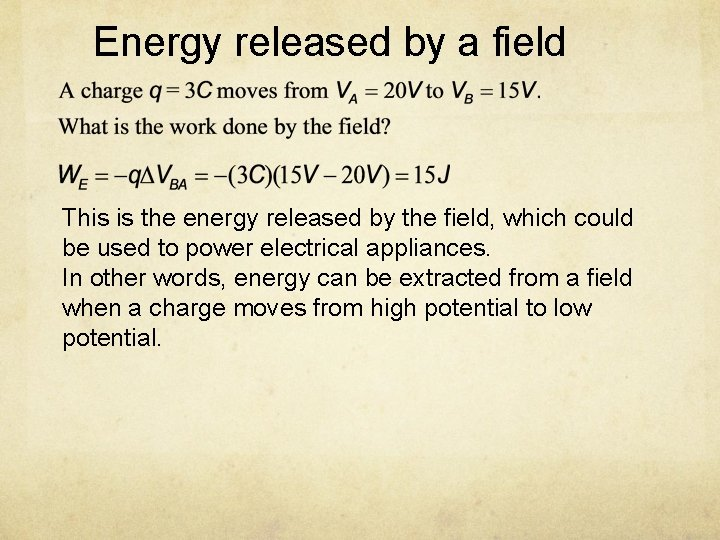Energy released by a field This is the energy released by the field, which could be used to power electrical appliances. In other words, energy can be extracted from a field when a charge moves from high potential to low potential.Potential at Infinity By convention, the electric potential at infinity is set to zero: Therefore, the change in KE for a charge released from infinity is given by: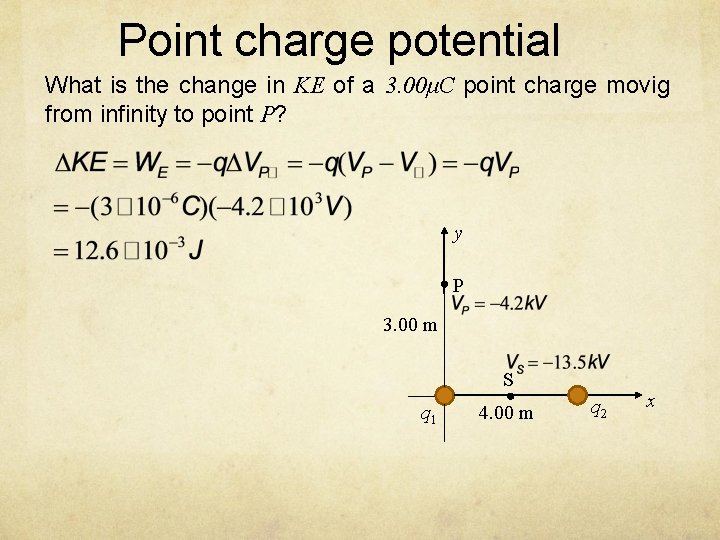Point charge potential What is the change in KE of a 3. 00μC point charge movig from infinity to point P? y P 3. 00 m S q 1 4. 00 m q 2 x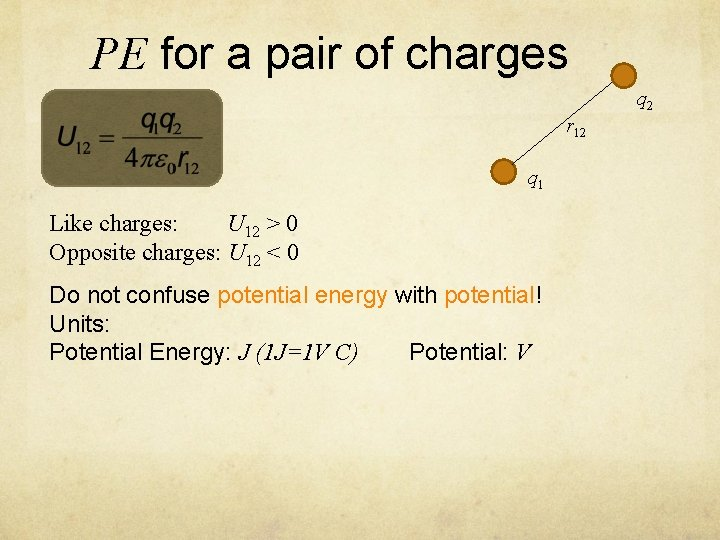PE for a pair of charges q 2 r 12 q 1 Like charges: U 12 > 0 Opposite charges: U 12 < 0 Do not confuse potential energy with potential! Units: Potential Energy: J (1 J=1 V C) Potential: VMultiple Charges Total potential energy obtained by calculating U for every pair of charges and add them up. In this case there are total of three pairs: q 1 r 13 q 3 r 12 q 2 r 23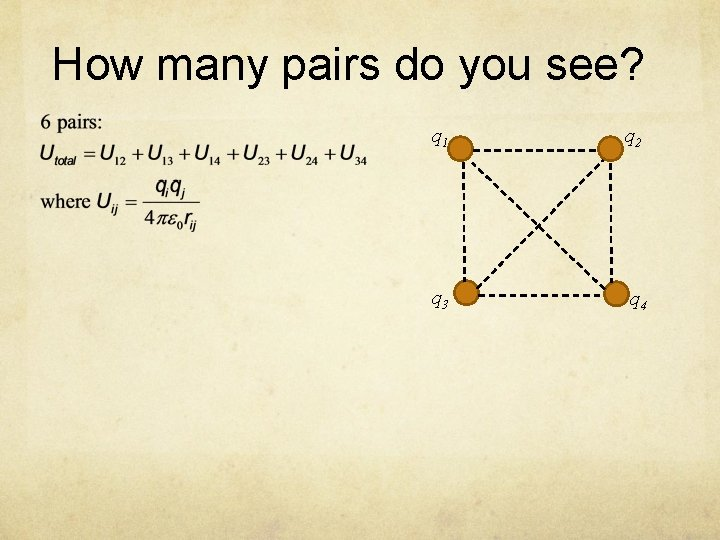How many pairs do you see? q 1 q 2 q 3 q 4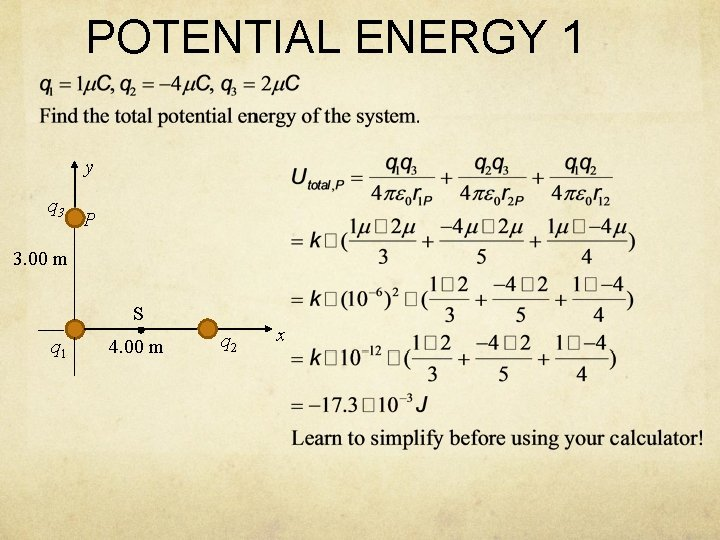POTENTIAL ENERGY 1 y q 3 P 3. 00 m S q 1 4. 00 m q 2 x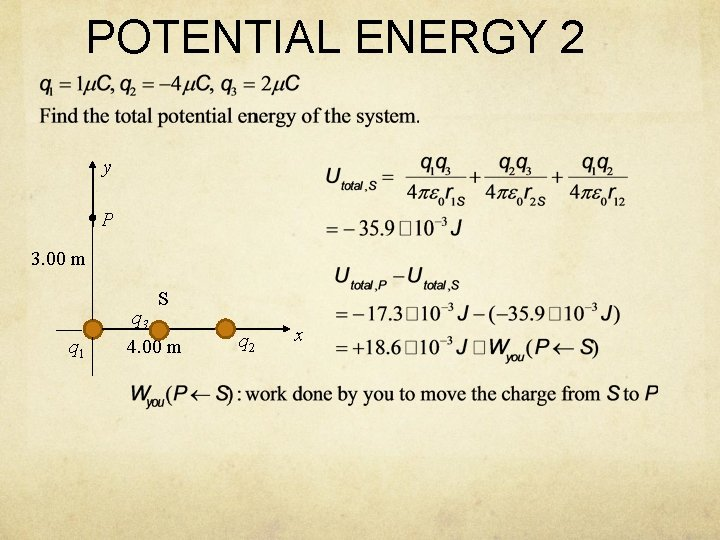POTENTIAL ENERGY 2 y P 3. 00 m S q 1 q 3 4. 00 m q 2 xIn greater detailsThe flow of charge If unrestrained: Positive charge flow from high V to low V Negative charge flow from low V to high V E field + Low V High V −Potential and Electric Field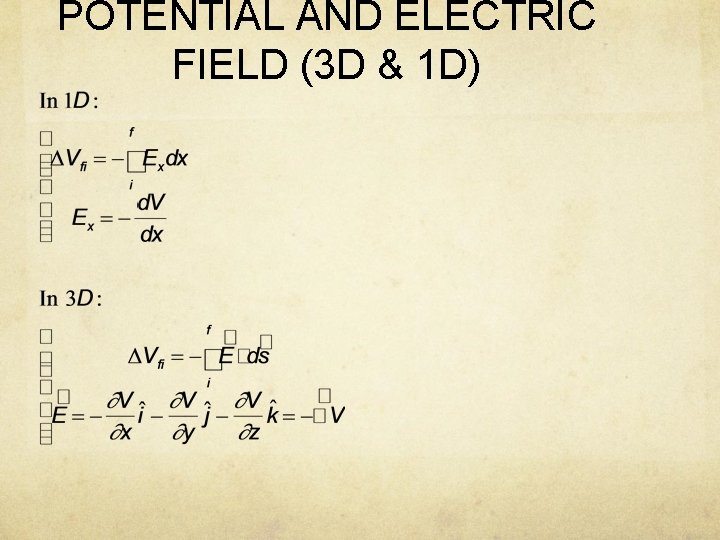POTENTIAL AND ELECTRIC FIELD (3 D & 1 D)Obtaining E from V The component of the electric field in a particular direction is the negative of the gradient of the potential in that direction. E field always point from high potential to low potential.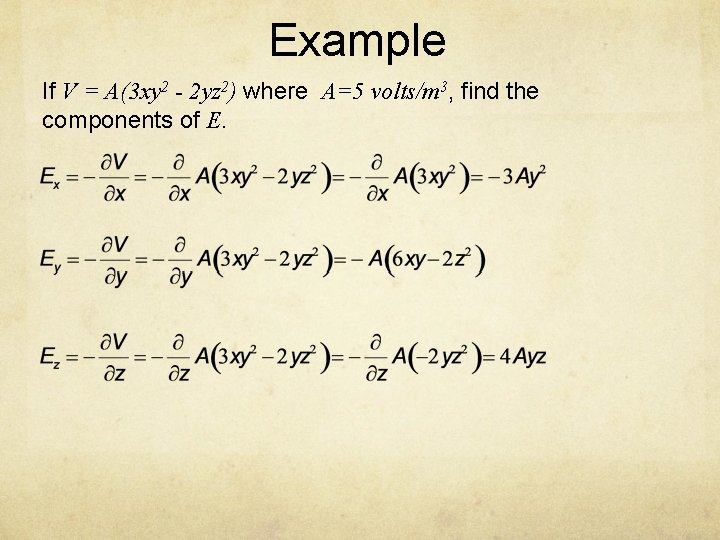Example If V = A(3 xy 2 - 2 yz 2) where A=5 volts/m 3, find the components of E.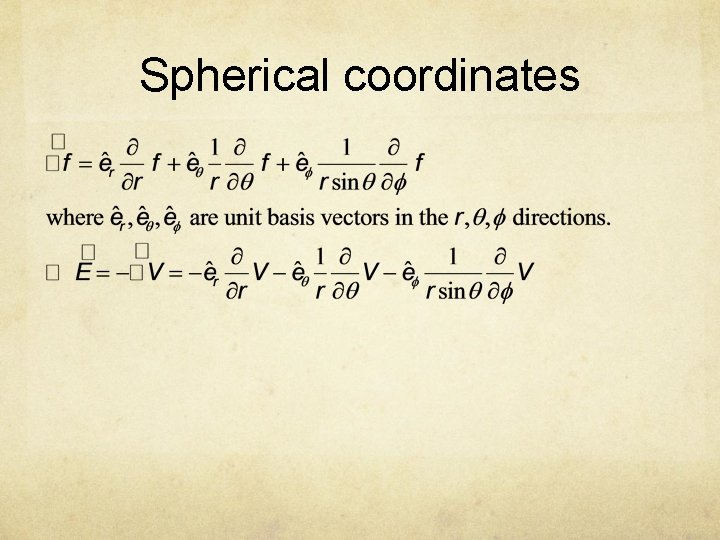Spherical coordinates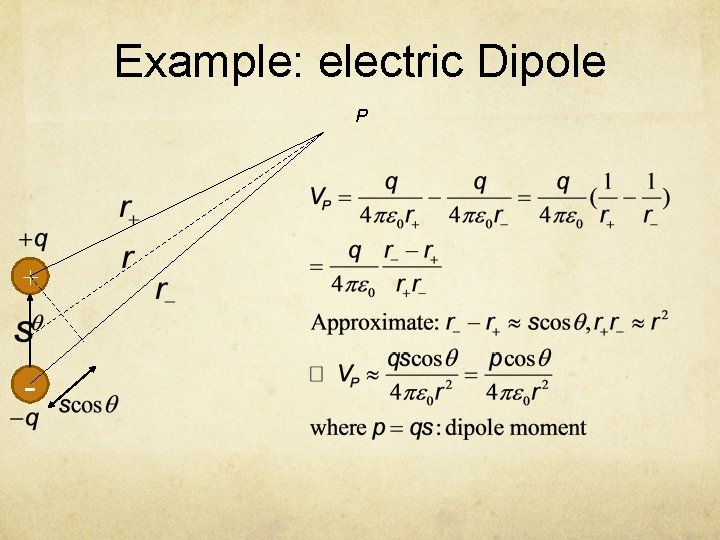Example: electric Dipole P + -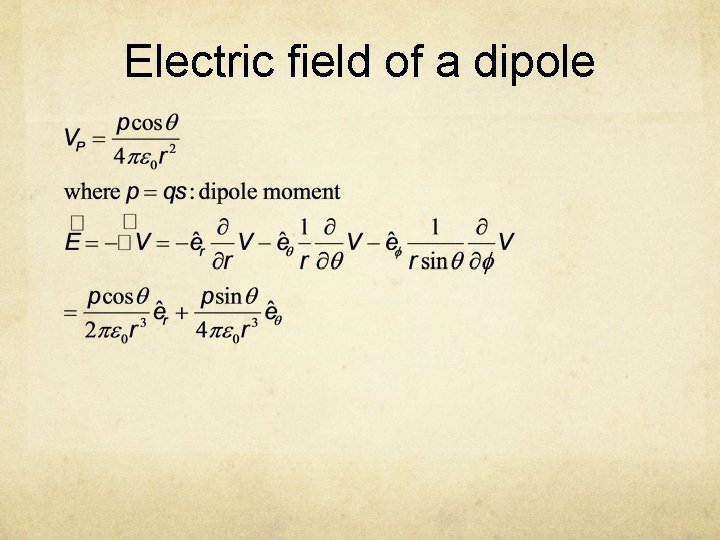Electric field of a dipole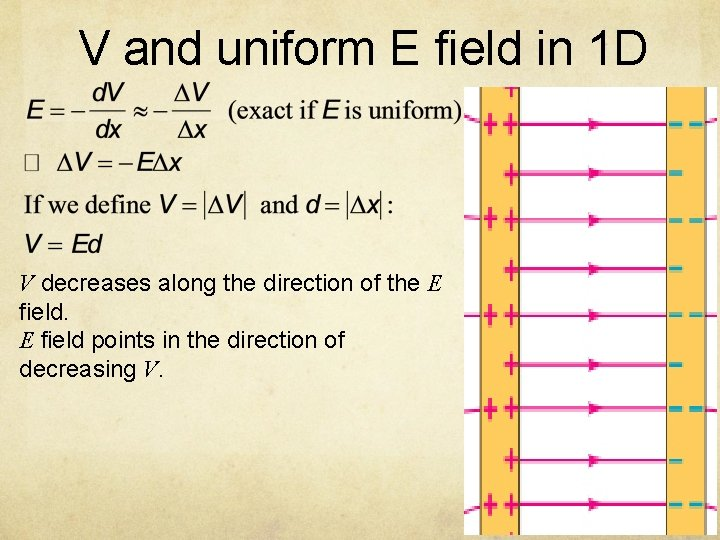V and uniform E field in 1 D V decreases along the direction of the E field points in the direction of decreasing V.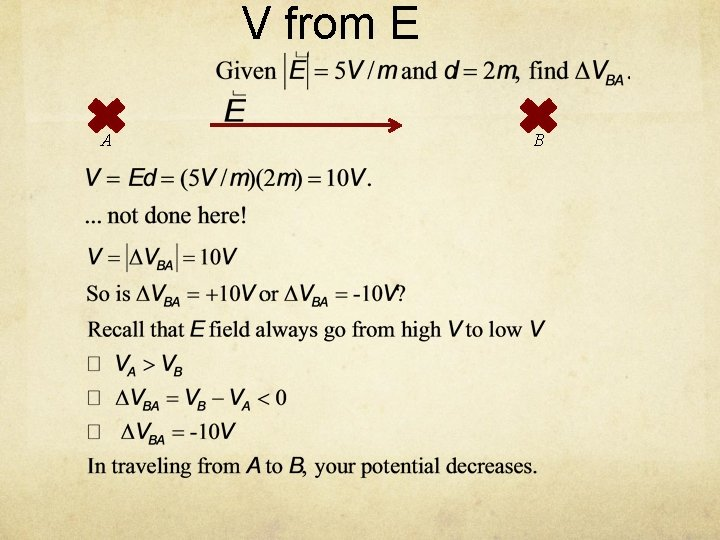V from E A B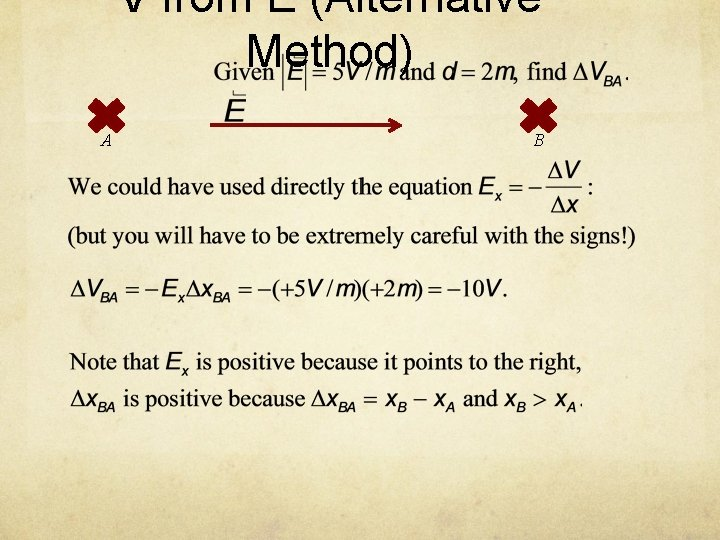V from E (Alternative Method) A B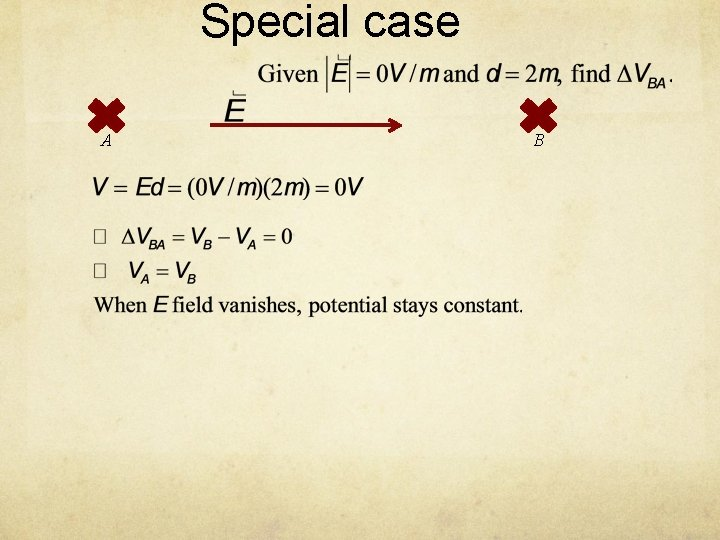Special case A BE from V A BE from V (Alternative Method) A BSpherically symmetric case For spherically symmetric charge distributions, the electric fields are radial and we have: The above equations are often combined with the convention of V∞=0 to give the potential: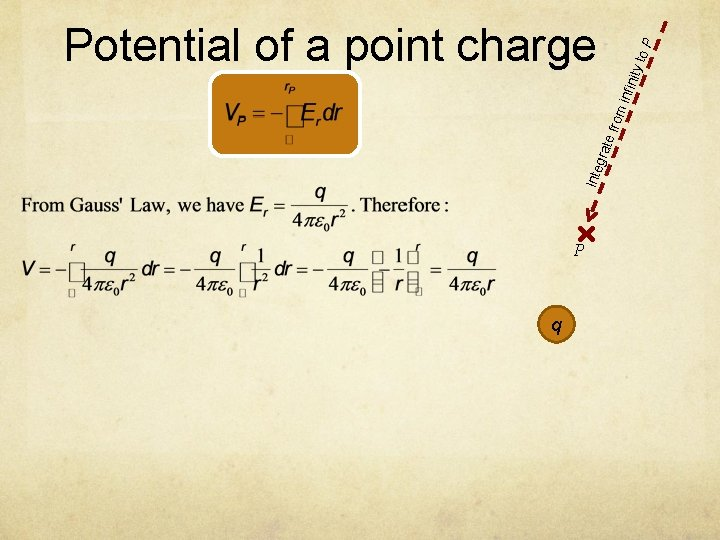Inte gra te f rom infin ity t o. P Potential of a point charge P q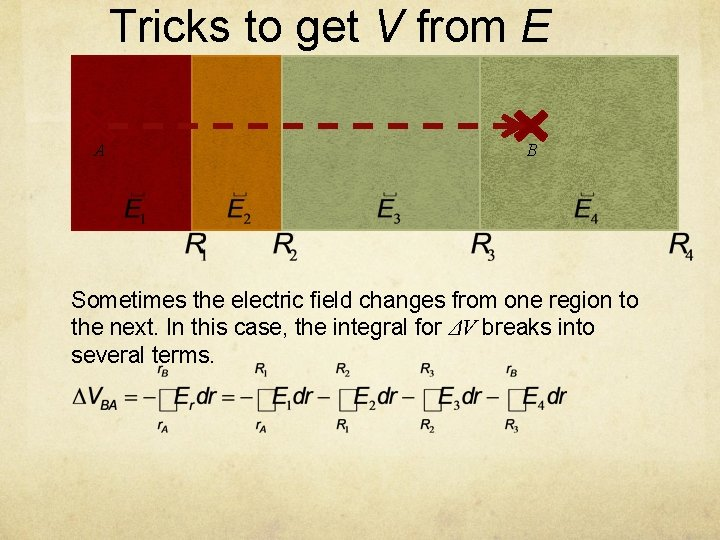Tricks to get V from E A B Sometimes the electric field changes from one region to the next. In this case, the integral for ΔV breaks into several terms.Potential of a uniform spherical charge distribution Suppose the sphere has a uniform volume charge density ρ, find potential inside and outside the sphere. R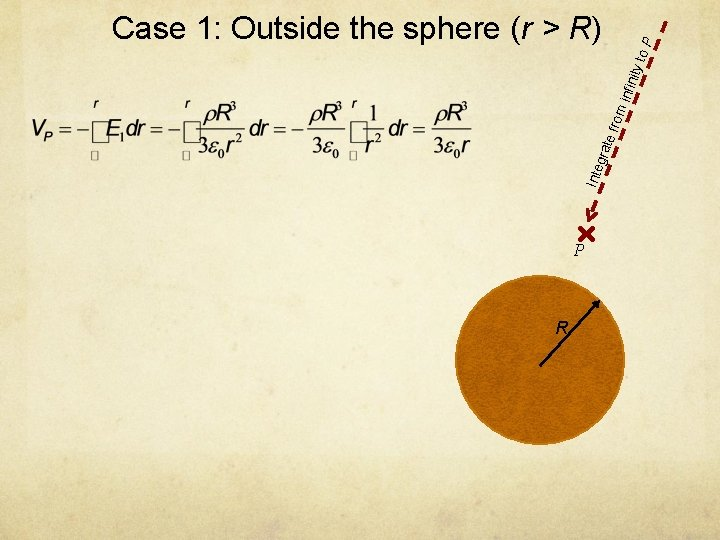Inte gra te f rom infin ity t o. P Case 1: Outside the sphere (r > R) P RInte gra te f rom infin ity t o. P Case 2: Inside the sphere (r < R) P R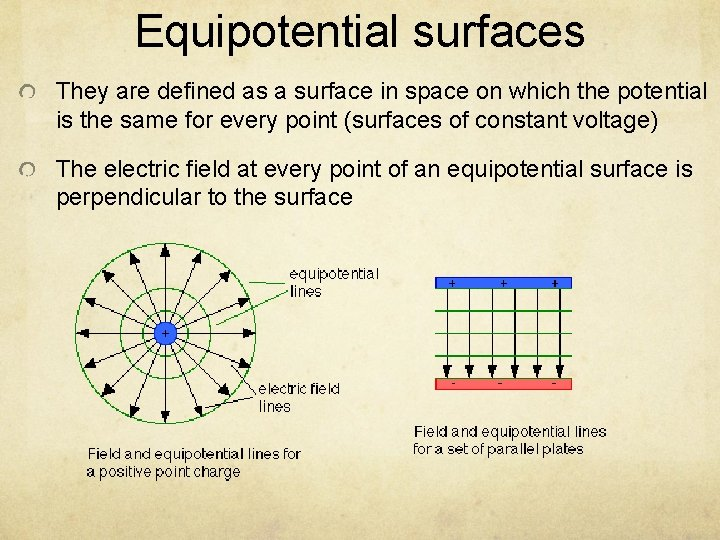Equipotential surfaces They are defined as a surface in space on which the potential is the same for every point (surfaces of constant voltage) The electric field at every point of an equipotential surface is perpendicular to the surface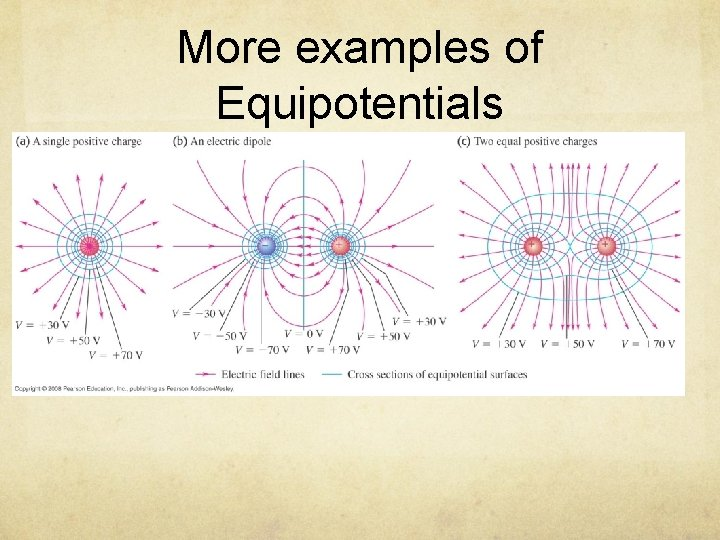More examples of Equipotentials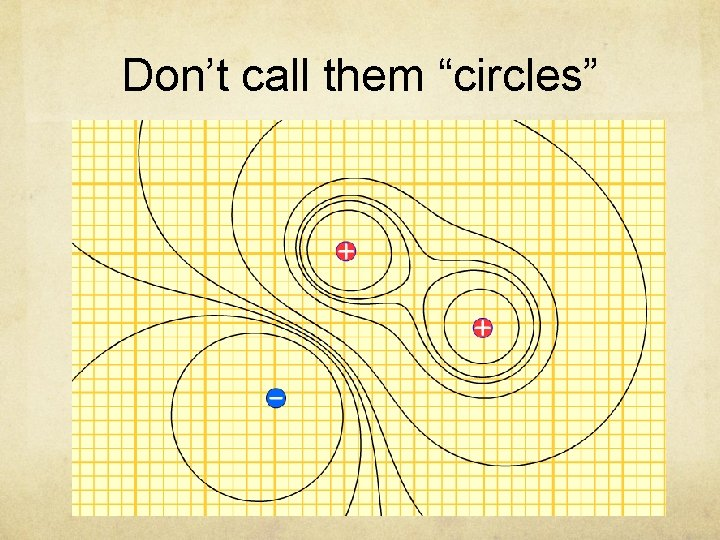Don’t call them “circles”Equipotential lines perpendicular to E field Consider the equation: To get zero, all line elements ds along the trace must be perpendicular to E.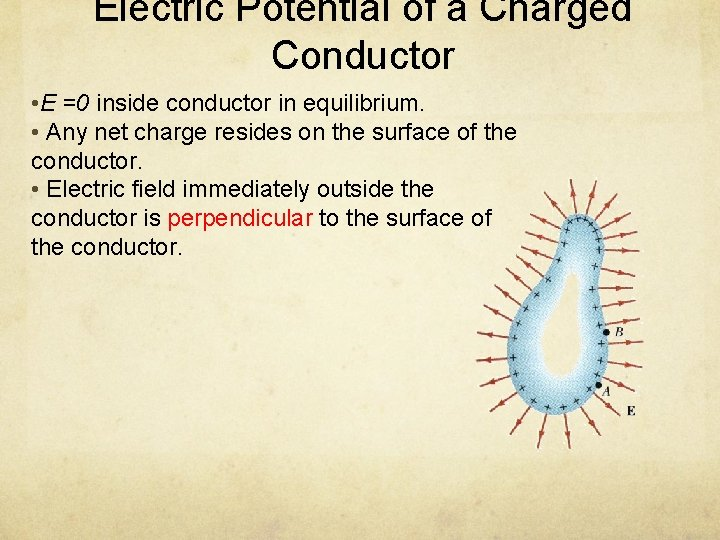Electric Potential of a Charged Conductor • E =0 inside conductor in equilibrium. • Any net charge resides on the surface of the conductor. • Electric field immediately outside the conductor is perpendicular to the surface of the conductor.Continuous charge distribution Find the electric potential at a point P located on the axis of a uniformly charged ring of radius a and a total charge Q. The plane of the ring is perpendicular to the x-axis. dq r a x P x Each charge element on the ring is the same distance from P, so r is a constant in the integration: